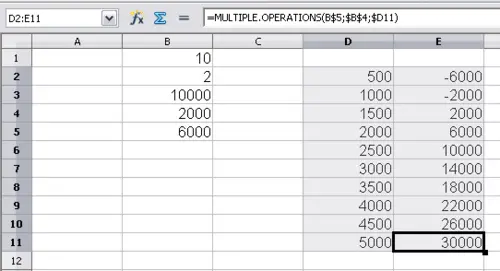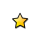On-line GuidesAll GuideseBook StoreiOS / AndroidLinux for BeginnersOffice ProductivityLinux InstallationLinux SecurityLinux UtilitiesLinux VirtualizationLinux KernelSystem/Network AdminProgrammingScripting LanguagesDevelopment ToolsWeb DevelopmentGUI Toolkits/DesktopDatabasesMail SystemsopenSolarisEclipse DocumentationTechotopia.comVirtuatopia.comAnswertopia.comHow To GuidesVirtualizationGeneral System AdminLinux SecurityLinux FilesystemsWeb ServersGraphics & DesktopPC HardwareWindowsProblem SolutionsPrivacy PolicyOpenOffice Calc 3.x Guide
Previous Page Home Next Page

## Multiple operations in columns or rows

In your spreadsheet, enter a formula to calculate a result from values that are stored in other cells. Then, set up a cell range where you enter some fixed values, and the Multiple Operations command will calculate the results depending on the formula.

In the Formula field, enter the cell reference to the formula that applies to the data range. In the Column input cell/Row input cell field, enter the cell reference to the corresponding cell that is part of the formula. This can be explained best by examples. (Cell references correspond to those in the following figures.)

Let’s say you produce toys that you sell for \$10 each (cell B1). Each toy costs \$2 to make (cell B2), in addition to which you have fixed costs of \$10,000 per year (cell B3). How much profit will you make in a year if you sell a particular number of toys?

### Calculating with one formula and one variable

1. To calculate the profit, first enter any number as the quantity (items sold); in this example, 2000 (cell B4). The profit is found from the formula Profit=Quantity * (Selling price – Direct costs) – Fixed costs. Enter this formula in B5: =B4*(B1-B2)-B3.
2. In column D enter given annual sales, one below the other; for example, 500 to 5000, in steps of 500.
3. Select the range D2:E11, and thus the values in column D and the empty cells alongside in column E.
4. Choose Data > Multiple Operations.
5. With the cursor in the Formulas field of the Multiple operations dialog, click cell B5.
6. Set the cursor in the Column input cell field and click cell B4. This means that B4, the quantity, is the variable in the formula, which is replaced by the selected column values. The figure below shows the worksheet and the Multiple operations dialog.
7. Click OK. The profits for the different quantities are now shown in column E.
8.Sheet showing results of multiple operations calculationsYou may find it easier to mark the required reference in the sheet if you click the Shrink icon to reduce the Multiple operations dialog to the size of the input field. The icon then changes to the Maximize icon; click it to restore the dialog to its original size.

### Calculating with several formulas simultaneously

1. In the sheet from the previous example, delete the contents of column E.
2. Enter the following formula in C5: =B5/B4. You are now calculating the annual profit per item sold.
3. Select the range D2:F11, thus three columns.
4. Choose Data > Multiple Operations.
5. With the cursor in the Formulas field of the Multiple operations dialog, select cells B5 thru C5.
6. Set the cursor in the Column input cell field and click cell B4. The figure below shows the worksheet and the Multiple operations dialog.
7. Click OK. Now the profits are listed in column E and the annual profit per item in column F. (You might want to format column F to show 2 decimal points, for easier comparison of the amounts.)

OpenOffice Calc 3.x Guide
Previous Page Home Next PagePublished under the terms of the Creative Commons License Design by Interspire